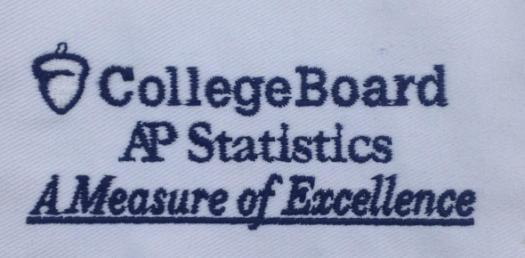# Ultimate Exam On AP Statistics! Trivia Quiz

5 Questions | Total Attempts: 635Settings.

Related Topics
• 1.
In a large high school, student leadership wants to randomly select a sample to survey the student body.  They will first randomly select 25 freshman, then randomly select 25 sophomores, then randomly select 25 juniors, and then randomly select 25 seniors.  This is an example of a
• A.

Cluster sample

• B.

Stratified sample

• C.

Simple random sample

• D.

Convenience sample

• E.

Systematic sample

• 2.
Below is a cumulative relative frequency plot (or, an ogive) for the scores of a large university class on a 90-point calculus exam.  Which of the following observations is correct?
• A.

The median score is at least 60 points.

• B.

The distribution of scores is skewed to the right.

• C.

The distribution of scores is skewed to the left.

• D.

The distribution of scores is roughly symmetric.

• E.

If a passing score is 60, most students passed the test.

• 3.
A least-squares regression line for predicting performance on a college entrance exam based on high school GPA is determined to be Predicted Score = 273.5 + 91.2(GPA).  One student in the study had a high school GPA of 3.0, and an exam score of 510.  What is the residual for this student?
• A.

26.2

• B.

43.9

• C.

-37.1

• D.

-26.2

• E.

37.1

• 4.
A recent poll reported that 43% of Californians approve of the job the Governor is doing, with a margin of sampling error of + or - 3.25% at a 95% level of confidence.  Which of these correctly interprets that margin of error?
• A.

There is a 3.2% chance that the proportion of all Californians who approve of the job the Governor is doing is not 43%.

• B.

There is a 95% chance that the proportion of all Americans who approve of the job the Governor is doing is 43%.

• C.

About 3.2% of all Californians approve of 95% of what the Governor does.

• D.

About 95% of polls conducted this way will give a sample proportion within 3.2 percentage points of the actual proportion of all Californians who approve of the job the Governor is doing.

• E.

About 95% of polls conducted in this way will find between 39.8% and 46.2% of those sampled approve of the job the Governor is doing.

• 5.
A test for heartworm in dogs shows a positive result in 96% of dogs that actually have heartworm, and shows negative results in 98% of dogs with no heartworm.  If heartworm actually occurs in 10% of dogs, what is the approximate probability that a randomly selected dog that tested positive for heartworm actually has heartworm?
• A.

11%

• B.

18%

• C.

84%

• D.

88%

• E.

96%